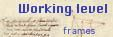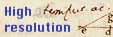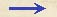Overview Level of Folio 156 rSize Height 302 mm, width 205 mm. Watermark Two watermarks. Crown, form 1. Thin crossbow. Drake's identification: Watermark type C14. Drake's description: Mountains 17 X 20mm. Date of earliest letter with this watermark is May 1608. Comments The folio is part of a larger sheet of paper combining the folios 156 and 157 . Contains calculations, drawings, minimal text.
 Folio 156 r (final text) C01 media inter ga au 184776 * 153072 = 369552 + 1293432 + 554328 + 923880 + 184776 = 28284031872 sqrt 28284031872 = 168178 C02 182576 * 2 = 365142 sqrt 36514200000 = 191087 C03 (191087 * 141422) : 200000 191087 * 141422 = 382174 + 382174 + 764348 + 191087 + 764348 + 191087 = 27023905714 27023905714 : 200000 = 135118 C04 16598 * 2 = 3319600000 3319600000 : 184776 = 17424Overview Level of Folio 156 r